a monstrous unimodular lattice

An integral $n$-dimensional lattice $L$ is the set of all integral linear combinations
$L = \mathbb{Z} \lambda_1 \oplus \dots \oplus \mathbb{Z} \lambda_n$
of base vectors $\{ \lambda_1,\dots,\lambda_n \}$ of $\mathbb{R}^n$, equipped with the usual (positive definite) inner product, satisfying
$(\lambda, \mu ) \in \mathbb{Z} \quad \text{for all \lambda,\mu \in \mathbb{Z}.}$
But then, $L$ is contained in its dual lattice $L^* = Hom_{\mathbb{Z}}(L,\mathbb{Z})$, and if $L = L^*$ we say that $L$ is unimodular.

If all $(\lambda,\lambda) \in 2 \mathbb{Z}$, we say that $L$ is an even lattice. Even unimodular lattices (such as the $E_8$-lattice or the $24$ Niemeier lattices) are wonderful objects, but they can only live in dimensions $n$ which are multiples of $8$.

Just like the Conway group $Co_0 = .0$ is the group of rotations of the Leech lattice $\Lambda$, one might ask whether there is a very special lattice on which the Monster group $\mathbb{M}$ acts faithfully by rotations. If such a lattice exists, it must live in dimension at least $196883$.Simon Norton (1952-2019) – Photo Credit

A first hint of such a lattice is in Conway’s original paper A simple construction for the Fischer-Griess monster group (but not in the corresponding chapter 29 of SPLAG).

Conway writes that Simon Norton showed ‘by a very simple computations that does not even require knowledge of the conjugacy classes, that any $198883$-dimensional representation of the Monster must support an invariant algebra’, which, after adding an identity element $1$, we now know as the $196884$-dimensional Griess algebra.

Further, on page 529, Conway writes:

Norton has shown that the lattice $L$ spanned by vectors of the form $1,t,t \ast t’$, where $t$ and $t’$ are transposition vectors, is closed under the algebra multiplication and integral with respect to the doubled inner product $2(u,v)$. The dual quotient $L^*/L$ is cyclic of order some power of $4$, and we believe that in fact $L$ is unimodular.

Here, transposition vectors correspond to transpositions in $\mathbb{M}$, that is, elements of conjugacy class $2A$.

In his post, Adam considers the $196883$-dimensional lattice $L’ = L \cap 1^{\perp}$ (which has $\mathbb{M}$ as its rotation symmetry group), and asks for the minimal norm (squared) of a lattice point, which he believes is $448$, and for the number of minimal vectors in the lattice, which might be
$2639459181687194563957260000000 = 9723946114200918600 \times 27143910000$
the number of oriented arcs in the Monster graph.

Here, the Monster graph has as its vertices the elements of $\mathbb{M}$ in conjugacy class $2A$ (which has $9723946114200918600$ elements) and with an edge between two vertices if their product in $\mathbb{M}$ again belongs to class $2A$, so the valency of the graph must be $27143910000$, as explained in that old post the monster graph and McKay’s observation.

When I asked Adam whether he had more information about his lattice, he kindly informed me that Borcherds told him that the Norton lattice $L$ didn’t turn out to be unimodular after all, but that a unimodular lattice with monstrous symmetry had been constructed by Scott Carnahan in the paper A Self-Dual Integral Form of the Moonshine Module.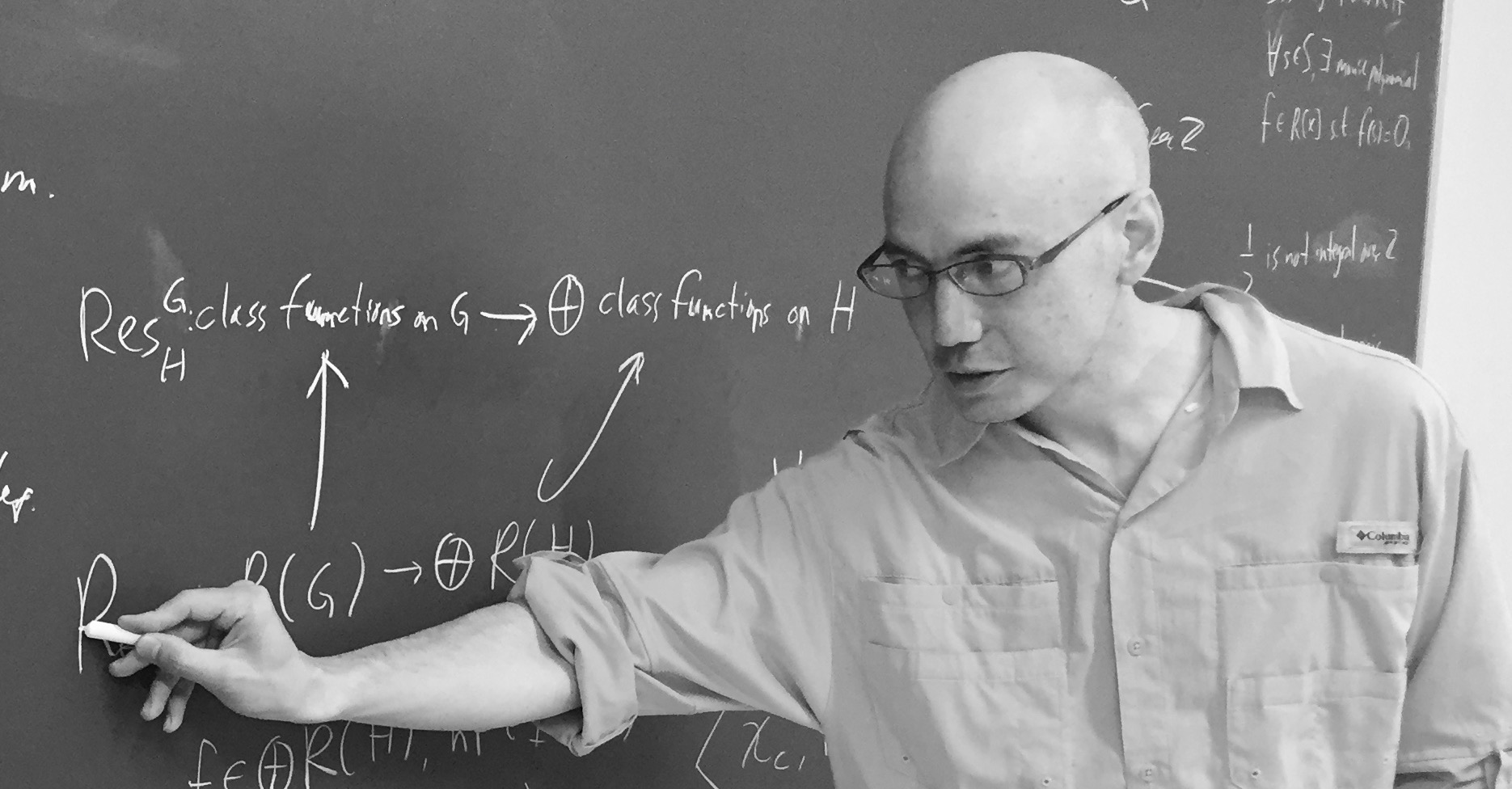Scott Carnahan – Photo Credit

The major steps (or better, the little bit of it I could grasp in this short time) in the construction of this unimodular $196884$-dimensional monstrous lattice might put a smile on your face if you are an affine scheme aficionado.

Already in his paper Vertex algebras, Kac-Moody algebras, and the Monster, Richard Borcherds described an integral form of any lattice vertex algebra. We’ll be interested in the lattice vertex algebra $V_{\Lambda}$ constructed from the Leech lattice $\Lambda$ and call its integral form $(V_{\Lambda})_{\mathbb{Z}}$.

One constructs the Moonshine module $V^{\sharp}$ from $V_{\Lambda}$ by a process called ‘cyclic orbifolding’, a generalisation of the original construction by Frenkel, Lepowsky and Meurman. In fact, there are now no less than 51 constructions of the moonshine module.

One starts with a fixed point free rotation $r_p$ of $\Lambda$ in $Co_0$ of prime order $p \in \{ 2,3,5,7,13 \}$, which one can lift to an automorphism $g_p$ of the vertex algebra $V_{\Lambda}$ of order $p$ giving an isomorphism $V_{\Lambda}/g_p \simeq V^{\sharp}$ of vertex operator algebras over $\mathbb{C}$.

For two distinct primes $p,p’ \in \{ 2,3,5,7,13 \}$ if $Co_0$ has an element of order $p.p’$ one can find one such $r_{pp’}$ such that $r_{pp’}^p=r_{p’}$ and $r_{pp’}^{p’}=r_p$, and one can lift $r_{pp’}$ to an automorphism $g_{pp’}$ of $V_{\Lambda}$ such that $V_{\Lambda}/g_{pp’} \simeq V_{\Lambda}$ as vertex operator algebras over $\mathbb{C}$.

Problem is that these lifts of automorphisms and the isomorphisms are not compatible with the integral form $(V_{\Lambda})_{\mathbb{Z}}$ of $V_{\Lambda}$, but ‘essentially’, they can be performed on
$(V_{\Lambda})_{\mathbb{Z}} \otimes_{\mathbb{Z}} \mathbb{Z}[\frac{1}{pp’},\zeta_{2pp’}]$
where $\zeta_{2pp’}$ is a primitive $2pp’$-th root of unity. These then give a $\mathbb{Z}[\tfrac{1}{pp’},\zeta_{2pp’}]$-form on $V^{\sharp}$.

Next, one uses a lot of subgroup information about $\mathbb{M}$ to prove that these $\mathbb{Z}[\tfrac{1}{pp’},\zeta_{2pp’}]$-forms of $V^{\sharp}$ have $\mathbb{M}$ as their automorphism group.

Then, using all his for different triples in $\{ 2,3,5,7,13 \}$ one can glue and use faithfully flat descent to get an integral form $V^{\sharp}_{\mathbb{Z}}$ of the moonshine module with monstrous symmetry and such that the inner product on $V^{\sharp}_{\mathbb{Z}}$ is positive definite.

Finally, one looks at the weight $2$ subspace of $V^{\sharp}_{\mathbb{Z}}$ which gives us our Carnahan’s $196884$-dimensional unimodular lattice with monstrous symmetry!

Beautiful as this is, I guess it will be a heck of a project to deduce even the simplest of facts about this wonderful lattice from running through this construction.

For example, what is the minimal length of vectors? What is the number of minimal length vectors? And so on. All info you might have is very welcome.

Know thy neighbours

Two lattices $L$ and $L’$ in the same vector space are called neighbours if their intersection $L \cap L’$ is of index two in both $L$ and $L’$.

In 1957, Martin Kneser gave a method to find all unimodular lattices (of the same dimension and signature) starting from one such unimodular lattice, finding all its neighbours, and repeating this with the new lattices obtained.

In other words, Kneser’s neighbourhood graph, with vertices the unimodular lattices (of fixed dimension and signature) and edges between them whenever the lattices are neighbours, is connected.Martin Kneser (1928-2004) – Photo Credit

Last time, we’ve constructed the Niemeier lattice $(A_1^{24})^+$ from the binary Golay code $\mathcal{C}_{24}$
$L = (A_1^{24})^+ = \mathcal{C}_{24} \underset{\mathbb{F}_2}{\times} (A_1^{24})^* = \{ \tfrac{1}{\sqrt{2}} \vec{v} ~|~\vec{v} \in \mathbb{Z}^{\oplus 24},~v=\vec{v}~mod~2 \in \mathcal{C}_{24} \}$
With hindsight, we know that $(A_1^{24})^+$ is the unique neighbour of the Leech lattice in the Kneser neighbourhood graph of the positive definite, even unimodular $24$-dimensional lattices, aka the Niemeier lattices.

Let’s try to construct the Leech lattice $\Lambda$ from $L=(A_1^{24})^+$ by Kneser’s neighbour-finding trick.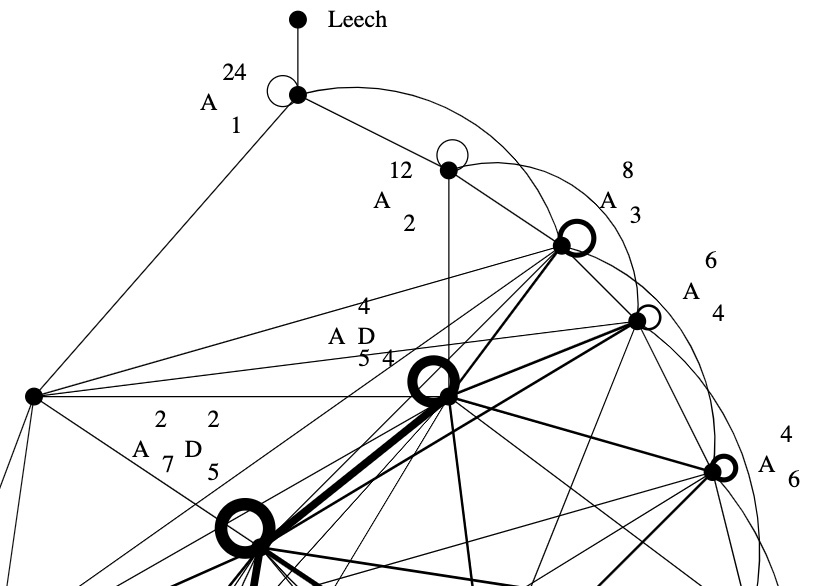Sublattices of $L$ of index two are in one-to-one correspondence with non-zero elements in $L/2L$. Take $l \in L – 2L$ and $m \in L$ such that the inner product $l.m$ is odd, then
$L_l = \{ x \in L~|~l.x~\text{is even} \}$
is an index two sublattice because $L = L_l \sqcup (L_l+m)$. By definition $l.x$ is even for all $x \in L_l$ and therefore $\frac{l}{2} \in L_l^*$. We have this situation
$L_l \subsetneq L = L^* \subsetneq L_l^*$
and $L_l^*/L_l \simeq \mathbb{F}_2 \oplus \mathbb{F}_2$, with the non-zero elements represented by $\{ \frac{l}{2}, m, \frac{l}{2}+m \}$. That is,
$L_l^* = L_l \sqcup (L_l+m) \sqcup (L_l+\frac{l}{2}) \sqcup (L_l+(\frac{l}{2}+m))$
This gives us three lattices
$\begin{cases} M_1 &= L_l \sqcup (L_l+m) = L \\ M_2 &= L_l \sqcup (L_l+\frac{l}{2}) \\ M_3 &= L_l \sqcup (L_l+(\frac{l}{2}+m)) \end{cases}$
and all three of them are unimodular because
$L_l \subsetneq M_i \subseteq M_i^* \subsetneq L_l^*$
and $L_l$ is of index $4$ in $L_l^*$.

Now, let’s assume the norm of $l$, that is, $l.l \in 4 \mathbb{Z}$. Then, either the norm of $\frac{l}{2}$ is odd (but then the norm of $\frac{l}{2}+m$ must be even), or the norm of $\frac{l}{2}$ is even, in which case the norm of $\frac{l}{2}+m$ is odd.

That is, either $M_2$ or $M_3$ is an even unimodular lattice, the other one being an odd unimodular lattice.

Let’s take for $l$ and $m$ the vectors $\lambda = \frac{1}{\sqrt{2}} (1,1,\dots,1) \in L – 2L$ and $\mu = \sqrt{2}(1,0,\dots,0) \in L$, then
$\lambda.\lambda = \frac{1}{2}\times 24 = 12 \quad \text{and} \quad \mu.\lambda = 1$
Because $\frac{\lambda}{2}.\frac{\lambda}{2} = \frac{12}{4}=3$ is odd, we have that
$\Lambda = L_{\lambda} \sqcup (L_{\lambda} + (\frac{\lambda}{2} + \mu))$
is an even unimodular lattice, which is the Leech lattice, and
$\Lambda_{odd} = L_{\lambda} \sqcup (L_{\lambda} + \frac{\lambda}{2})$
is an odd unimodular lattice, called the odd Leech lattice.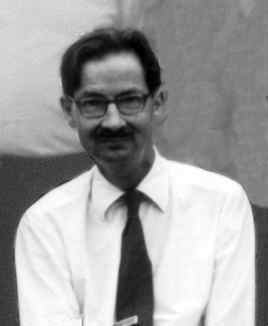John Leech (1926-1992) – Photo Credit

Let’s check that these are indeed the Leech lattices, meaning that they do not contain roots (vectors of norm two).

The only roots in $L = (A_1^{24})^+$ are the $48$ roots of $A_1^{24}$ and they are of the form $\pm \sqrt{2} [ 1, 0^{23} ]$, but none of them lies in $L_{\lambda}$ as their inproduct with $\lambda$ is one. So, all non-zero vectors in $L_{\lambda}$ have norm $\geq 4$.

As for the other part of $\Lambda$ and $\Lambda_{odd}$
$(L_{\lambda} + \frac{\lambda}{2}) \sqcup (L_{\lambda} + \mu + \frac{\lambda}{2}) = (L_{\lambda} \sqcup (L_{\lambda}+\mu))+\frac{\lambda}{2} = L + \frac{\lambda}{2}$
From the description of $L=(A_1^{24})^+$ it follows that every coordinate of a vector in $L + \frac{\lambda}{2}$ is of the form
$\frac{1}{\sqrt{2}}(v+\frac{1}{2}) \quad \text{or} \quad \frac{1}{\sqrt{2}}(v+\frac{3}{2})$
with $v \in 2 \mathbb{Z}$, with the second case instances forming a codeword in $\mathcal{C}_{24}$. In either case, the square of each of the $24$ coordinates is $\geq \frac{1}{8}$, so the norm of such a vector must be $\geq 3$, showing that there are no roots in this region either.

If one takes for $l$ a vector of the form $\frac{1}{\sqrt{2}} v = \frac{1}{\sqrt{2}}[1^a,0^{24-a}]$ where $a=8,12$ or $16$ and $v \in \mathcal{C}_{24}$, takes $m=\mu$ as before, and repeats the construction, one gets the other Niemeier-neighbours of $(A_1^{24})^+$, that is, the lattices $(A_2^{12})^+$, $(A_3^8)^+$ and $(D_4^6)^+$.

For $a=12$ one needs a slightly different argument, see section 0.2 of Richard Borcherds’ Ph.D. thesis.

The Leech lattice neighbour

Here’s the upper part of Kneser‘s neighbourhood graph of the Niemeier lattices: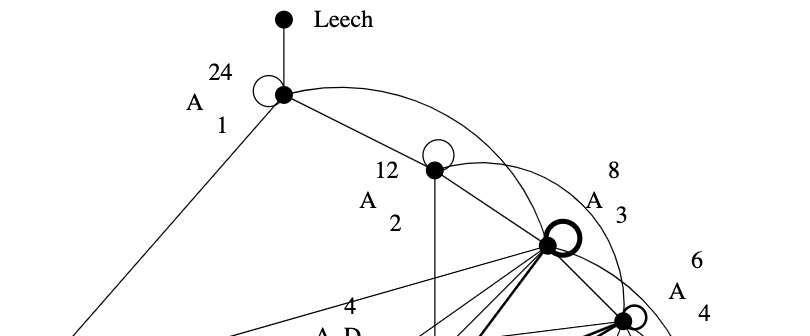The Leech lattice has a unique neighbour, that is, among the $23$ remaining Niemeier lattices there is a unique one, $(A_1^{24})^+$, sharing an index two sub-lattice with the Leech.

How would you try to construct $(A_1^{24})^+$, an even unimodular lattice having the same roots as $A_1^{24}$?

The root lattice $A_1$ is $\sqrt{2} \mathbb{Z}$. It has two roots $\pm \sqrt{2}$, determinant $2$, its dual lattice is $A_1^* = \tfrac{1}{\sqrt{2}} \mathbb{Z}$ and we have $A_1^*/A_1 \simeq C_2 \simeq \mathbb{F}_2$.

Thus, $A_1^{24}= \sqrt{2} \mathbb{Z}^{\oplus 24}$ has $48$ roots, determinant $2^{24}$, its dual lattice is $(A_1^{24})^* = \tfrac{1}{\sqrt{2}} \mathbb{Z}^{\oplus 24}$ and the quotient group $(A_1^{24})^*/A_1^{24}$ is $C_2^{24}$ isomorphic to the additive subgroup of $\mathbb{F}_2^{\oplus 24}$.

A larger lattice $A_1^{24} \subseteq L$ of index $k$ gives for the dual lattices an extension $L^* \subseteq (A_1^{24})^*$, also of index $k$. If $L$ were unimodular, then the index has to be $2^{12}$ because we have the situation
$A_1^{24} \subseteq L = L^* \subseteq (A_1^{24})^*$
So, Kneser’s glue vectors form a $12$-dimensional subspace $\mathcal{C}$ in $\mathbb{F}_2^{\oplus 24}$, that is,
$L = \mathcal{C} \underset{\mathbb{F}_2}{\times} (A_1^{24})^* = \{ \tfrac{1}{\sqrt{2}} \vec{v} ~|~\vec{v} \in \mathbb{Z}^{\oplus 24},~v=\vec{v}~mod~2 \in \mathcal{C} \}$
Because $L = L^*$, the linear code $\mathcal{C}$ must be self-dual meaning that $v.w = 0$ (in $\mathbb{F}_2$) for all $v,w \in \mathcal{C}$. Further, we want that the roots of $A_1^{24}$ and $L$ are the same, so the minimal number of non-zero coordinates in $v \in \mathcal{C}$ must be $8$.

That is, $\mathcal{C}$ must be a self-dual binary code of length $24$ with Hamming distance $8$.Marcel Golay (1902-1989) – Photo Credit

We now know that there is a unique such code, the (extended) binary Golay code, $\mathcal{C}_{24}$, which has

• one vector of weight $0$
• $759$ vectors of weight $8$ (called ‘octads’)
• $2576$ vectors of weight $12$ (called ‘dodecads’)
• $759$ vectors of weight $16$
• one vector of weight $24$

The $759$ octads form a Steiner system $S(5,8,24)$ (that is, for any $5$-subset $S$ of the $24$-coordinates there is a unique octad having its non-zero coordinates containing $S$).

Witt constructed a Steiner system $S(5,8,24)$ in his 1938 paper “Die $5$-fach transitiven Gruppen von Mathieu”, so it is not unthinkable that he checked the subspace of $\mathbb{F}_2^{\oplus 24}$ spanned by his $759$ octads to be $12$-dimensional and self-dual, thereby constructing the Niemeier-lattice $(A_1^{24})^+$ on that sunday in 1940.

John Conway classified all nine self-dual codes of length $24$ in which the weight
of every codeword is a multiple of $4$. Each one of these codes $\mathcal{C}$ gives a Niemeier lattice $\mathcal{C} \underset{\mathbb{F}_2}{\times} (A_1^{24})^*$, all but one of them having more roots than $A_1^{24}$.

Vera Pless and Neil Sloan classified all $26$ binary self-dual codes of length $24$.

Witt and his Niemeier lattices

Sunday, January 28th 1940, Hamburg

Ernst Witt wants to get two papers out of his system because he knows he’ll have to enter the Wehrmacht in February.

The first one, “Spiegelungsgruppen und Aufzählung halbeinfacher Liescher Ringe”, contains his own treatment of the root systems of semisimple Lie algebras and their reflexion groups, following up on previous work by Killing, Cartan, Weyl, van der Waerden and Coxeter.(Photo: Natascha Artin, Nikolausberg 1933): From left to right: Ernst Witt; Paul Bernays; Helene Weyl; Hermann Weyl; Joachim Weyl, Emil Artin; Emmy Noether; Ernst Knauf; unknown woman; Chiuntze Tsen; Erna Bannow (later became wife of Ernst Witt)

Important for our story is that this paper contains the result stating that integral lattices generated by norm 2 elements are direct sums of root systems of the simply laced Dynkin diagrans $A_n, D_n$ and $E_6,E_7$ or $E_8$ (Witt uses a slightly different notation).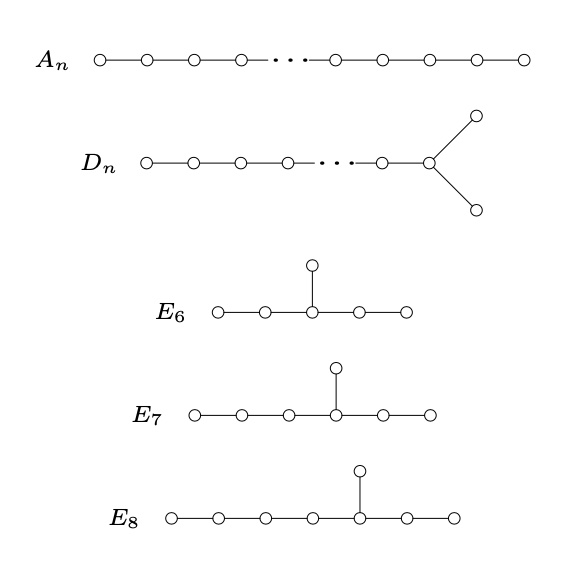In each case, Witt knows of course the number of roots and the determinant of the Gram matrix
$\begin{array}{c|cc} & \# \text{roots} & \text{determinant} \\ \hline A_n & n^2+n & n+1 \\ D_n & 2n^2-2n & 4 \\ E_6 & 72 & 3 \\ E_7 & 126 & 2 \\ E_8 & 240 & 1 \end{array}$
The second paper “Eine Identität zwischen Modulformen zweiten Grades” proves that there are just two positive definite even unimodular lattices (those in which every squared length is even, and which have one point per unit volume, that is, have determinant one) in dimension sixteen, $E_8 \oplus E_8$ and $D_{16}^+$. Previously, Louis Mordell showed that the only unimodular even lattice in dimension $8$ is $E_8$.

The connection with modular forms is via their theta series, listing the number of lattice points of each squared length
$\theta_L(q) = \sum_{m=0}^{\infty} \#\{ \lambda \in L : (\lambda,\lambda)=m \} q^{m}$
which is a modular form of weight $n/2$ ($n$ being the dimension which must be divisible by $8$) in case $L$ is a positive definitive even unimodular lattice.

The algebra of all modular forms is generated by the Eisenstein series $E_2$ and $E_3$ of weights $4$ and $6$, so in dimension $8$ we have just one possible theta series
$\theta_L(q) = E_2^2 = 1+480 q^2+ 61920 q^4+ 1050240 q^6+ \dots$

It is interesting to read Witt’s proof of his main result (Satz 3) in which he explains how he constructed the possible even unimodular lattices in dimension $16$.

He knows that the sublattice of $L$ generated by the $480$ norm two elements must be a direct sum of root lattices. His knowledge of the number of roots in each case tells him there are only two possibilities
$E_8 \oplus E_8 \qquad \text{and} \qquad D_{16}$
The determinant of the Gram matrix of $E_8 \oplus E_8$ is one, so this one is already unimodular. The remaining possibility
$D_{16} = \{ (x_1,\dots,x_{16}) \in \mathbb{Z}^{16}~|~x_1+ \dots + x_{16} \in 2 \mathbb{Z} \}$
has determinant $4$ so he needs to add further lattice points (necessarily contained in the dual lattice $D_{16}^*$) to get it unimodular. He knows the coset representatives of $D_{16}^*/D_{16}$:
$\begin{cases} =(0, \dots,0) &~\text{of norm 0} \\ =(\tfrac{1}{2},\dots,\tfrac{1}{2}) &~\text{of norm 4} \\ =(0,\dots,0,1) &~\text{of norm 1} \\ =(\tfrac{1}{2},\dots,\tfrac{1}{2},-\tfrac{1}{2}) &~\text{of norm 4} \end{cases}$
and he verifies that the determinant of $D_{16}^+=D_{16}+(+D_{16})$ is indeed one (btw. adding coset $$ gives an isomorphic lattice). Witt calls this procedure to arrive at the correct lattices forced (‘zwangslaufig’).

So, how do you think Witt would go about finding even unimodular lattices in dimension $24$?

To me it is clear that he would start with a direct sum of root lattices whose dimensions add up to $24$, compute the determinant of the Gram matrix and, if necessary, add coset classes to arrive at a unimodular lattice.

Today we would call this procedure ‘adding glue’, after Martin Kneser, who formalised this procedure in 1967.

On January 28th 1940, Witt writes that he found more than $10$ different classes of even unimodular lattices in dimension $24$ (without giving any details) and mentioned that the determination of the total number of such lattices will not be entirely trivial (‘scheint nicht ganz leicht zu sein’).

The complete classification of all $24$ even unimodular lattices in dimension $24$ was achieved by Hans Volker Niemeier in his 1968 Ph.D. thesis “Definite quadratische Formen der Dimension 24 und Diskriminante 1”, under the direction of Martin Kneser. Naturally, these lattices are now known as the Niemeier lattices.

Which of the Niemeier lattices were known to Witt in 1940?

There are three obvious certainties: $E_8 \oplus E_8 \oplus E_8$, $E_8 \oplus D_{16}^+$ (both already unimodular, the second by Witt’s work) and $D_{24}^+$ with a construction analogous to the one of $D_{16}^+$.

To make an educated guess about the remaining Witt-Niemeier lattices we can do two things:

1. use our knowledge of Niemeier lattices to figure out which of these Witt was most likely to stumble upon, and
2. imagine how he would adapt his modular form approach in dimension $16$ to dimension $24$.

Here’s Kneser’s neighbourhood graph of the Niemeier lattices. Its vertices are the $24$ Niemeiers and there’s an edge between $L$ and $M$ whenever the intersection $L \cap M$ is of index $2$ in both $L$ and $M$. In this case, $L$ and $M$ are called neighbours.Although the theory of neighbours was not known to Witt, the graph may give an indication of how likely it is to dig up a new Niemeier lattice by poking into an already discovered one.
The three certainties are the three lattices at the bottom of the neighborhood graph, making it more likely for the lattices in the lower region to be among Witt’s list.

For the other approach, the space of modular forms of weight $12$ is two dimensional, with a basis formed by the series
$\begin{cases} E_6(q) = 1 + \tfrac{65520}{691}(q+2049 q^2 + 177148 q^3+4196353q^4+\dots \\ \Delta(q) = q-24 q^2+252q^3-1472q^4+ \dots \end{cases}$

If you are at all with me, Witt would start with a lattice $R$ which is a direct sum of root lattices, so he would know the number of its roots (the norm $2$ vectors in $R$), let’s call this number $r$. Now, he wants to construct an even unimodular lattice $L$ containing $R$, so the theta series of both $L$ and $R$ must start off with $1 + r q^2 + \dots$. But, then he knows
$\theta_L(q) = E_6(q) + (r-\frac{65520}{691})\Delta(q)$
and comparing coefficients of $\theta_L(q)$ with those of $\theta_R(q)$ will give him an idea what extra vectors he has to throw in.

If we’re generous to Witt (and frankly, why shouldn’t we), we may believe that he used his vast knowledge of Steiner systems (a few years earlier he wrote the definite paper on the Mathieu groups, and a paper on Steiner systems) to construct in this way the lattices $(A_1^{24})^+$ and $(A_2^{12})^+$.

The ‘glue’ for $(A_1^{24})^+$ is coming from the extended binary Golay code, which itself uses the Steiner system $S(5,8,24)$. $(A_2^{12})^+$ is constructed using the extended ternary Golay code, based on the Steiner system $S(5,6,12)$.

The one thing that would never have crossed his mind that sunday in 1940 was to explore the possibility of an even unimodular 24-dimensional lattice $\Lambda$ without any roots!

One with $r=0$, and thus with a theta series starting off as
$\theta_{\Lambda}(q) = 1 + 196560 q^4 + 16773120 q^6 + \dots$
No, he did not find the Leech lattice that day.

If he would have stumbled upon it, it would have simply blown his mind.

It would have been so much against all his experiences and intuitions that he would have dropped everything on the spot to write a paper about it, or at least, he would have mentioned in his ‘more than $10$ lattices’-claim that, surprisingly, one of them was an even unimodular lattice without any roots.

Who discovered the Leech lattice?

The Leech lattice was, according to wikipedia, ‘originally discovered by Ernst Witt in 1940, but he did not publish his discovery’ and it ‘was later re-discovered in 1965 by John Leech’. However, there is very little evidence to support this claim.

The facts

What is certain is that John Leech discovered in 1965 an amazingly dense 24-dimensional lattice ${\Lambda}$ having the property that unit balls around the lattice points touch, each one of them having exactly 196560 neighbors. The paper ‘Notes on sphere packings’ appeared in 1967 in the Canad. J. Math. 19, 251-267.

Compare this to the optimal method to place pennies on a table, leading to the hexagonal tiling, each penny touching exactly 6 others. Similarly, in dimension 8 the densest packing is the E8 lattice in which every unit ball has exactly 240 neighbors.

The Leech lattice ${\Lambda}$ can be characterized as the unique unimodular positive definite even lattice such that the length of any non-zero vector is at least two.

The list of all positive definite even unimodular lattices, ${\Gamma_{24}}$, in dimension 24 was classified later by Hans-Volker Niemeier and are now known as the 24 Niemeier lattices.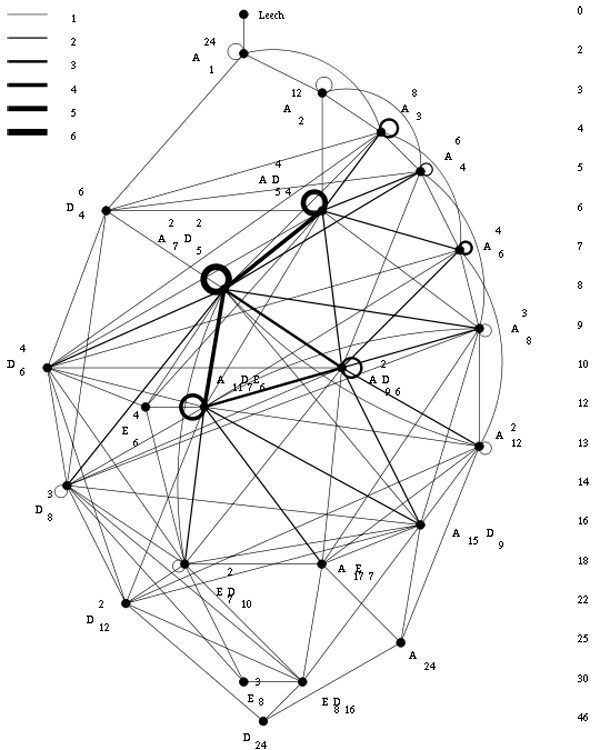For the chronology below it is perhaps useful to note that, whereas Niemeier’s paper did appear in 1973, it was submitted april 5th 1971 and is just a minor rewrite of Niemeier’s Ph.D. “Definite quadratische Formen der Dimension 24 und Diskriminante 1” obtained in 1968 from the University of Göttingen with advisor Martin Kneser.

The claim

On page 328 of Ernst Witt’s Collected Papers Ina Kersten recalls that Witt gave a colloquium talk on January 27, 1970 in Hamburg entitled “Gitter und Mathieu-Gruppen” (Lattices and Mathieu-groups). In this talk Witt claimed to have found nine lattices in ${\Gamma_{24}}$ as far back as 1938 and that on January 28, 1940 he found two additional lattices ${M}$ and ${\Lambda}$ while studying the Steiner system ${S(5,8,24)}$.

On page 329 of the collected papers is a scan of the abstract Witt wrote in the colloquium book in Bielefeld where he gave a talk “Uber einige unimodularen Gitter” (On certain unimodular lattices) on January 28, 1972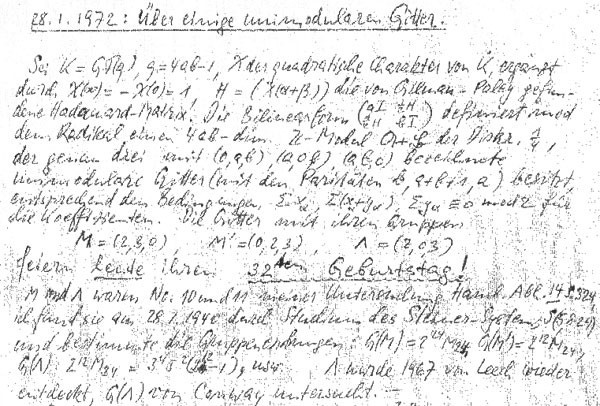Here, Witt claims that he found three new lattices in ${\Gamma_{24}}$ on January 28, 1940 as the lattices ${M}$, ${M’}$ and ${\Lambda}$ ‘feiern heute ihren 32sten Gebursttag!’ (celebrate today their 32nd birthday).

He goes on telling that the lattices ${M}$ and ${\Lambda}$ were number 10 and 11 in his list of lattices in ${\Gamma_{24}}$ in his paper “Eine Identität zwischen Modulformen zweiten Grades” in the Abh. Math. Sem. Univ. Hamburg 14 (1941) 323-337 and he refers in particular to page 324 of that paper.

He further claims that he computed the orders of their automorphism groups and writes that ${\Lambda}$ ‘wurde 1967 von Leech wieder-entdeckt’ (was re-discovered by Leech in 1967) and that its automorphism group ${G(\Lambda)}$ was studied by John Conway. Recall that Conway’s investigations of the automorphism group of the Leech lattice led to the discovery of three new sporadic groups, the Conway groups ${Co_1,Co_2}$ and ${Co_3}$.

However, Witt’s 1941-paper does not contain a numbered list of 24-dimensional lattices. In fact, apart from ${E_8+E_8+E_8}$ is does not contain a single lattice in ${\Gamma_{24}}$. The only relevant paragraph is indeed on page 324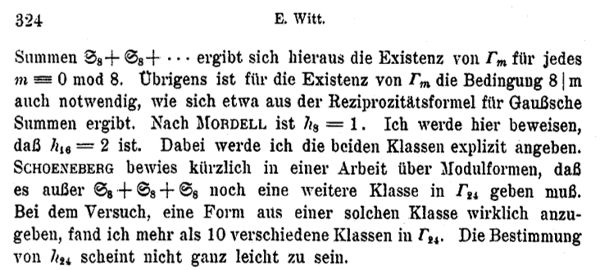He observes that Mordell already proved that there is just one lattice in ${\Gamma_8}$ (the ${E_8}$-lattice) and that the main result of his paper is to prove that there are precisely two even unimodular 16-dimensional lattices : ${E_8+E_8}$ and another lattice, now usually called the 16-dimensional Witt-lattice.

He then goes on to observe that Schoeneberg knew that ${\# \Gamma_{24} > 1}$ and so there must be more lattices than ${E_8+E_8+E_8}$ in ${\Gamma_{24}}$. Witt concludes with : “In my attempt to find such a lattice, I discovered more than 10 lattices in ${\Gamma_{24}}$. The determination of ${\# \Gamma_{24}}$ does not seem to be entirely trivial.”

Hence, it is fair to assume that by 1940 Ernst Witt had discovered at least 11 of the 24 Niemeier lattices. Whether the Leech lattice was indeed lattice 11 on the list is anybody’s guess.

Next time we will look more closely into the historical context of Witt’s 1941 paper.

Published
Categorized as stories